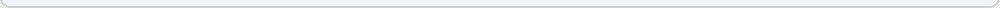# Compound Interest Calculator

Compound Interest in its most simple form is where you have money in an interest bearing investment such as a savings account or money market account, where you keep interest distributions in the account, thus increasing (compounding) future interest distributions. This is our simplest calculator and it can tell you how your money will grow over time when left alone in one of these investments. This calculator will compound your interest monthly like most savings accounts.

 Interest Rate: % (the current interest rate, such as 2) Starting Value: \$ (value in dollars of your account) Years to hold: (the number of years for which you want to calculate results)